### python – 内存管理

1.0 python引用机制

1.1 python动态类型

2.0 python引用计数

2.1 引用计数器原理

2.2 获取引用计数（getrefcount）

2.3 增加引用计数

2.4 减少引用计数

2.5 循环引用的情况

2.5.1 内存泄露:

2.5.2 内存溢出：（out of memory）

3.0 python垃圾回收

3.1 gc机制

3.2 三种情况触发垃圾回收

3.3 分代(generation)回收

3.4 标记清除

4.0 python 缓冲池

4.1   整数对象缓冲池

4.2  字符串缓存

4.3  python内存管理总结

5.0 深拷贝与浅拷贝

1.浅拷贝只会拷贝第一层地址

2.深拷贝会拷贝每一层的值

# 1.0 python引用机制

## 1.1 python动态类型

• 我们在程序中写的对象名，只是指向这一对象的引用(reference)

• 引用和对象分离，是动态类型的核心

• 引用可以随时指向一个新的对象（内存地址会不一样）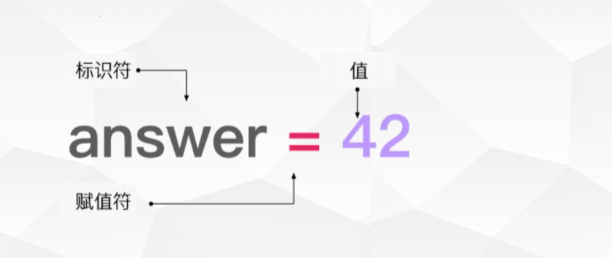#########################################

## 2.0 python引用计数

#########################################

## 2.1 引用计数器原理

#########################################

## 2.2 获取引用计数（getrefcount）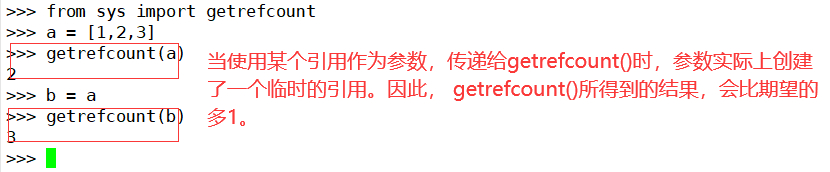#########################################

## 2.3 增加引用计数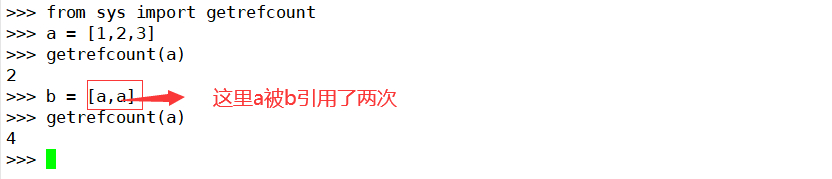#########################################

## 2.4 减少引用计数

del删除或者重新引用时候，引用计数会变化（del只是删除引用）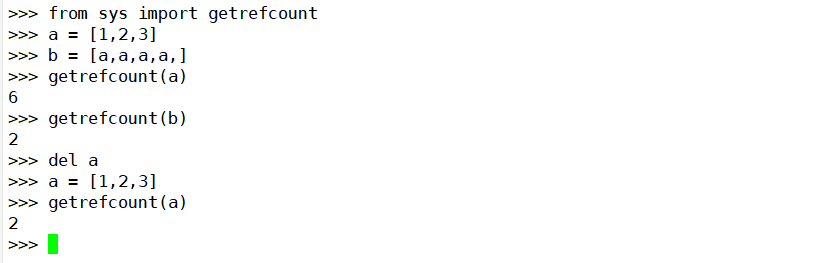#########################################

## 2.5 循环引用的情况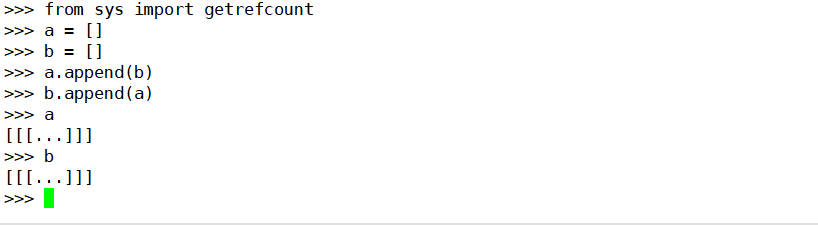#########################################

### 2.5.1 内存泄露:

#########################################

### 2.5.2 内存溢出：（out of memory）

#########################################

# 3.0 python垃圾回收

#########################################

## 3.1 gc机制

GC作为现代编程语言的自动内存管理机制，专注于两件事

• 找到内存中无用的垃圾资源

• 清除这些垃圾并把内存让出来给其他对象使用。

GC彻底把程序员从资源管理的重担中解放出来，让他们有更多的时间放 在业务逻辑上。但这并不意味

#########################################

## 3.2 三种情况触发垃圾回收

1.调用gc.collect()

2.gc达到阈值

3.程序退出时候

#########################################

## 3.3 分代(generation)回收

• Python将所有的对象分为0，1，2三代。

• 所有的新建对象都是0代对象。

• 当某一代对象经历过垃圾回收，依然存活，那么它就被归入下一代对象。

• 垃圾回收启动时，一定会扫描所有的0代对象。

• 如果0代经过一定次数垃圾回收，那么就启动对0代和1代的扫描清理。

• 当1代也经历了一定次数的垃圾回收后，那么会启动对0，1，2，即对所有对象进行扫描

#########################################

## 3.4 标记清除

1. 标记：活动（有被引用）, 非活动（可被删除）

2. 清除：清除所有非活动的对象

#########################################

# 4.0 python 缓冲池

## 4.1   整数对象缓冲池

``a = 200b = 200getrefcount(a)5getrefcount(b)5id(a)140116833235072id(b)140116833235072>>> a = 1>>> b = 1>>> getrefcount(a)873>>> getrefcount(b)873>>> id(a)140596260116384>>> id(b)140596260116384>>> a = 257>>> b = 257>>> getrefcount(a)2>>> getrefcount(b)2>>> id(a)140596125739920>>> id(b)140596125740048``

#########################################

## 4.2  字符串缓存

 注意：

``   str1 = abcstr2 = abcid(str1)140116834714936id(str2)140116834714936str1 is str2Truestr1 = abc str2 = abc str1 == str2Truestr1 is str2False``

`` str1  = #str2  = #str1 is str2True``

`` str1 = a*20str2 = a*20str1 is str2Truestr1 = a*21str2 = a*21str1 is str2False``

#########################################

## 4.3  python内存管理总结

#########################################

# 5.0 深拷贝与浅拷贝

#########################################

## 1.浅拷贝只会拷贝第一层地址

``d1{'a': [1, 2], 'b': 2}d2 = d1.copy()d2{'a': [1, 2], 'b': 2}d1 is d2Falsed1['a'][1, 2]d1['a'].append(3)d1{'a': [1, 2, 3], 'b': 2}d2{'a': [1, 2, 3], 'b': 2}id(di['a'])Traceback (most recent call last):File <stdin>, line 1, in <module>NameError: name 'di' is not definedid(d1['a'])140116834121224id(d2['a'])140116834121224``

#########################################

## 2.深拷贝会拷贝每一层的值

import copy

copy.deepcopy()

``  import copy d5 = d3.copy()d5{'a': [1, 2, 3, 4], 'b': 2}d6 = copy.deepcopy(d5)d6{'a': [1, 2, 3, 4], 'b': 2}d5 is d6Falsed5['a'].append(5)d5{'a': [1, 2, 3, 4, 5], 'b': 2}d6{'a': [1, 2, 3, 4], 'b': 2}``

#########################################